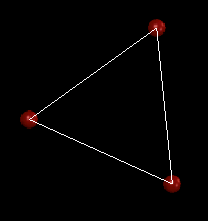# FlatRedBall.Math.Geometry.Polygon.Points

## Introduction

The Points property is an array of Point values which contain the position of each point on the polygon. These values are in “object space”. In other words, they are relative to the containing Polygon’s position and rotation values.

The Points property can be assigned – this essentially changes the shape of the Polygon. This is a more-efficient and often more-convenient way to change a Polygon rather than to construct a new one if your game requires dynamic shapes. For more information, see this section.

## Code Example – Setting Points

``` // First create the Polygon

// Create the Point array.  These points are relative to the center
// of the Polygon.
Point[] pointArray =
{
new Point(-1,  1), // top left
new Point( 1,  1), // top right
new Point( 1, -1), // bottom right
new Point(-1, -1), // bottom left
new Point(-1,  1)  // repeat top left to close Polygon
};

// Set the points
myPolygon.Points = pointArray;
```

## Code Example – Getting absolute Point positions

The values in the Points array are all relative to the containing Polygon. This means that if the Polygon moves or rotates, the values stored in the Points property will remain the same.

To retrieve the absolute position of each Point, the relative points must be converted into world (or absolute) coordinates.

The following code creates a Polygon which spins automatically and is repositioned based off of Keyboard activity. Three Sprites are positioned in the world coordinates of each of the Polygon’s points in the Update method. Since the Sprites are being positioned in world space, the position of the points must be converted to world space so that the Sprites appear in the proper position.

```using Microsoft.Xna.Framework;
using FlatRedBall.Math.Geometry;
using FlatRedBall.Input;
```

Add the following at class scope:

```SpriteList spriteList = new SpriteList();
Polygon polygon;
```

Add the following in Initialize after initializing FlatRedBall:

``` int numberOfSides = 3;

polygon = Polygon.CreateEquilateral(numberOfSides, 5, 0);
polygon.RotationZVelocity = .5f;

for (int i = 0; i < numberOfSides; i++)
{
sprite.ScaleX = sprite.ScaleY = .5f;
}
```

``` InputManager.Keyboard.ControlPositionedObject(polygon);

Matrix rotationMatrix = polygon.RotationMatrix;

for (int i = 0; i < spriteList.Count; i++)
{
// First get the point in the polygon's object space
spriteList[i].Position = new Vector3(
(float)polygon.Points[i].X,
(float)polygon.Points[i].Y,
0);

// Apply the rotation
FlatRedBall.Math.MathFunctions.TransformVector(
ref spriteList[i].Position, ref rotationMatrix);

// Now add the polygon's position
spriteList[i].Position += polygon.Position;
}
```